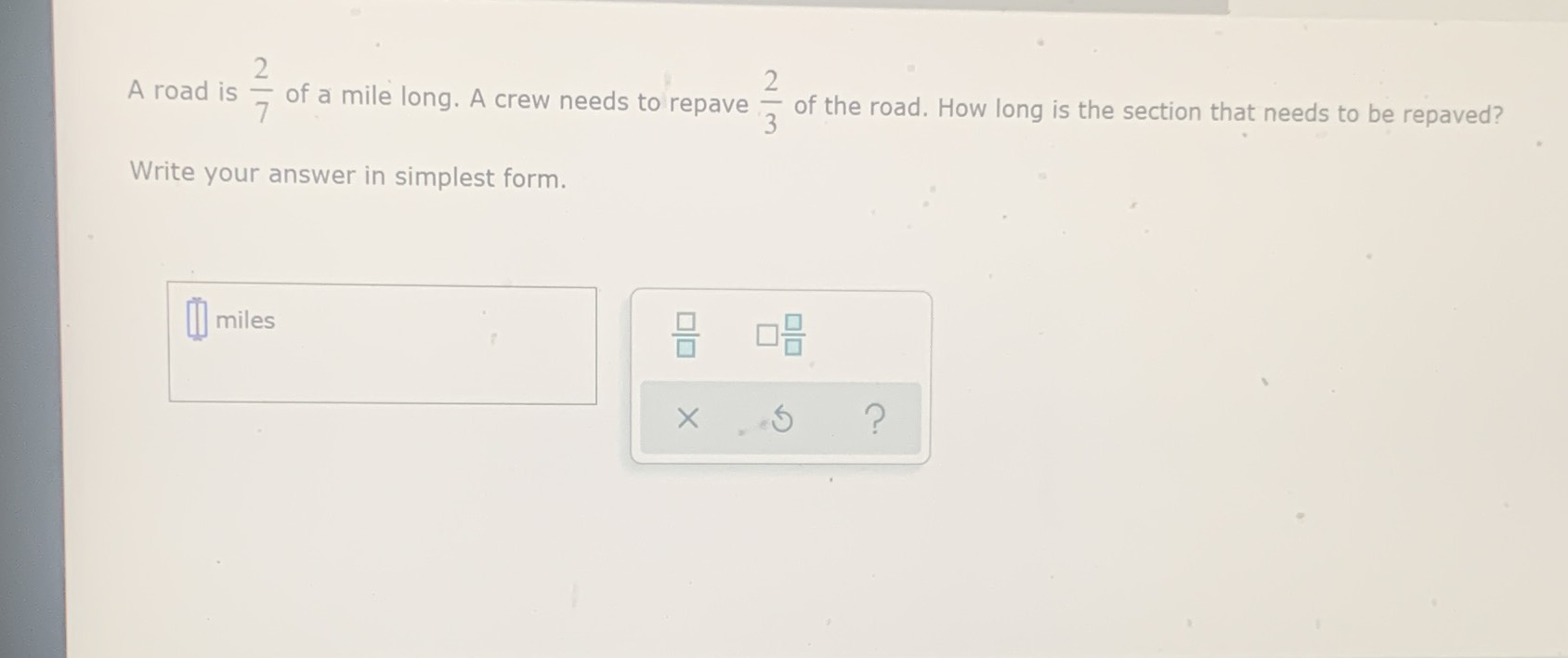### ¿Todavía tienes preguntas de matemáticas?

Pregunte a nuestros tutores expertos
Algebra
PreguntaA road is $$\frac { 2 } { 7 }$$ of a mile long. A crew needs to repave $$\frac { 2 } { 3 }$$ of the road. How long is the section that needs to be repaved? Write your answer in simplest form.

$$\frac{4}{21}$$